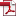A Joint Resource Allocation Scheme for Relay Enhanced Multi-cell Orthogonal Frequency Division Multiple Networks
• KSII Transactions on Internet and Information Systems
Monthly Online Journal (eISSN: 1976-7277)

# A Joint Resource Allocation Scheme for Relay Enhanced Multi-cell Orthogonal Frequency Division Multiple Networks

Vol. 7, No. 2, February 25, 201310.3837/tiis.2013.02.007, Download Paper (Free):#### Abstract

This paper formulates resource allocation for decode-and-forward (DF) relay assisted multi-cell orthogonal frequency division multiple (OFDM) networks as an optimization problem taking into account of inter-cell interference and users fairness. To maximize the transmit rate of system we propose a joint interference coordination, subcarrier and power allocation algorithm. To reduce the complexity, this semi-distributed algorithm divides the primal optimization into three sub-optimization problems, which transforms the mixed binary nonlinear programming problem (BNLP) into standard convex optimization problems. The first layer optimization problem is used to get the optimal subcarrier distribution index. The second is to solve the problem that how to allocate power optimally in a certain subcarrier distribution order. Based on the concept of equivalent channel gain (ECG) we transform the max-min function into standard closed expression. Subsequently, with the aid of dual decomposition, water-filling theorem and iterative power allocation algorithm the optimal solution of the original problem can be got with acceptable complexity. The third sub-problem considers dynamic co-channel interference caused by adjacent cells and redistributes resources to achieve the goal of maximizing system throughput. Finally, simulation results are provided to corroborate the proposed algorithm.